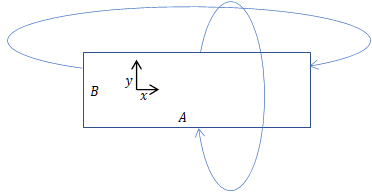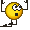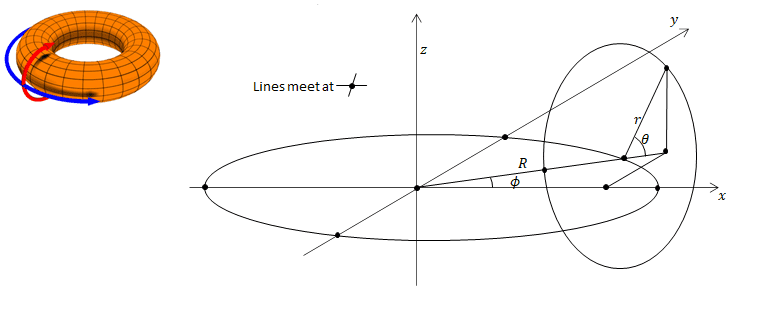# Is a three-torus maximally symmetric? Does curvature determine volume?

Gold Member
TL;DR Summary
Is it true that in three Riemannian dimensions that the curvature scalar determines whether the volume is finite or infinite? Is a flat three-torus a counter example? How do you describe a flat three-torus?
Spatial slices of the Robertson-Walker metrics are maximally symmetric so they must have a constant curvature. Is it true that in three Riemannian dimensions that a constant curvature scalar determines whether the volume is finite or infinite? Carroll seems to have given a counter-example for the flat case when the metric is Euclidean. That could be R³ or, he says, it could be a "more complicated manifold, such as a three-torus ##S^1\times S^1\times S^1##." I know that a two-torus does not have constant curvature so it is not maximally symmetric. I doubt if a three-torus does better. Do we think Carroll meant a "flat three-torus", but surely that would not be ##S^1\times S^1\times S^1##. What would it be?

Here is my simple definition of a flat two-torus:It is like a rectangle size ##A\times B## with coordinates ##x,y## but if you go off an edge, you come back to the opposite edge, so adding multiples of ##A## to the ##x## coordinate of ##B## to the ##y## coordinate keep you in the same place. It's a bit like a torus ...

A torus is intrinsically flat. Identifying boundaries of a compact flat manifold does not introduce (intrinsic) curvature.

Summary:: Is it true that in three Riemannian dimensions that the curvature scalar determines whether the volume is finite or infinite? Is a flat three-torus a counter example? How do you describe a flat three-torus?
The answer to your first question is simply no, and your flat three torus example is a suitable counterexample. To make your initial statement true, topological criteria must be added (and I am not prepared to state them with technical precision). Similar counterexamples exist for negative curvature - finite volume negative curvature manifolds can be constructed.

One statement that may be true (I am not sure of all technicalities) is that if all sectional curvatures are positive everywhere and all bounded below (greater than some value), then the manifold cannot be infinite.

Last edited:
Staff Emeritus
Interesting question. The cut-and-paste the edges together argument suggests that considered as an affine space, the torus is flat. I recall from some previous discussions that it is possible to calculate curvature in an affine space, a space that has a connection but no metric, though it's not something I know by experience how to do, exactly. I'm sure it'd be very educational to learn, but - a lot of work.

However, a cylinder is well known to be flat - cutting and pasting the edges together doesn't change the fact that you can parallel transport a vector around a closed path on the cylinder without rotating it.

So the torus is basically the same thing, but we have to glue two pairs of edges together, not just one. I suspect we might not be able to embed a flat torus in a 3d space with a metric. But my intuition suggests that we could do it by embedding the torus in a 4d space. We use the third dimension to roll up the flat piece of paper into a cylinder, and we use the fourth spatial dimension to roll up the cylinder so we can paste it's edges together.

This isn't a very rigorous argument, unfortunately, so it could be wrong in some important detail. It's probably best to explore curvature in affine spaces than to construct an embedding.

A candidate embedding for a cylinder would be a 3d line element dx^2 + dy^2 + dx^2, with a constraint of dx^2 + dy^2 = constant, and a candidate embedding for a torus would be the 4d line element dx^2 + dy^2 + dz^2 + dw^2 , with two constraints dx^2 + dy^2 = constant, and dz^2 + dw^2 = constant. But it's not obvious to me how to calculate the intrinsic curvature of the resulted embedded surface.

A flat 2-torus can definitely be smoothly embedded in 4d Euclidean space, with induced metric being everywhere the the standard Euclidean 2d flat metric, but the smooth embedding is not possible in 3D Euclidean space.

•Nugatory
Staff Emeritus
A flat 2-torus can definitely be smoothly embedded in 4d Euclidean space, with induced metric being everywhere the the standard Euclidean 2d flat metric, but the smooth embedding is not possible in 3D Euclidean space.

I thought about it some more and can demonstrate the first part now. Let the 4d metric being dx^2 + dy^2 + dz^2 + dw^2, and set dx^2 + dy^2=1 and dw^2+dz^2=1. Let w,x be the coordinates. Then the induced line element turns out to be:

$$\frac{dw^2}{1-w^2} + \frac{dx^2}{1-x^2}$$

Running this line element through GRTensor confirms it's flat.

The induced line element comes from ##y=\sqrt{1-x^2}## ##z=\sqrt{1-w^2}## and
$$dx^2 + dy^2 + dz^2 + dw^2 = dw^2\left(1+\left( \frac{dz}{dw} \right)^2 \right) + dx^2\left(1+\left( \frac{dy}{dx} \right)^2 \right)$$

•George Keeling
@pervect , Try using polar coordinates for the torus in the xy plane, and in the zw plane. You will be amused at how much simpler the calculation is. You directly end up with a Euclidean metric in two angles with two scale factors for the two radii, for the induced metric. Then, a further trivial scaling coordinate transform brings you exactly to the standard Euclidean 2 metric.

•George Keeling
Gold Member
I was baffled by @pervect although I could follow the maths*. I couldn't see how his equations mapped onto my flat torus. @PAllen solved that. I think that his polar coordinates give me$$x=A\cos{\theta}$$$$y=A\sin{\theta}$$$$z=B\cos{\phi}$$$$w=B\sin{\phi}$$they induce a constant metric$${ds}^2=A^2{d\theta}^2+B^2{d\phi}^2$$and a constant metric gives zero curvature immediately.

Now I can see why this is like a flat torus. Adding ##2\pi## to ##\theta## or ##\phi## takes you back to the same place in 4D just like my diagram of the flat torus where I started. And we have used the two circles (##S^1##'s) in 4D to get to this manifold which explains why Carroll was saying that a three-torus was ##S^1\times S^1\times S^1## and was flat. I suppose he just started in six dimensions.

Thanks very much all for thinking while I slept!*PS It was easy to calculate the zero curvature from pervect's description because I have found a wonderful formula for the Ricci tensor of a symmetric metric from K. Z. Win at https://arxiv.org/pdf/gr-qc/9602015v1.pdf. Diagonal components are given by
$$4R_{\mu\mu}=\left(\partial_\mu\ln{\left|g_{\mu\mu}\right|}-2\partial_\mu\right)\partial_\mu\ln{\left|\frac{g}{g_{\mu\mu}}\right|}-\sum_{\sigma\neq\mu}\left[\left(\partial_\mu\ln{\left|g_{\sigma\sigma}\right|}\right)^2+\left(\partial_\sigma\ln{\frac{\left|g\right|}{{g_{\mu\mu}}^2}}+2\partial_\sigma\right)\left(g^{\sigma\sigma}\partial_\sigma g_{\mu\mu}\right)\right]$$The partial sum is over ##n## coordinate components and in this case the expression disappears immediately. No need to calculate Christoffel or Riemann components.I was baffled by @pervect although I could follow the maths*. I couldn't see how his equations mapped onto my flat torus. @PAllen solved that. I think that his polar coordinates give me$$x=A\cos{\theta}$$$$y=A\sin{\theta}$$$$z=B\cos{\phi}$$$$w=B\sin{\phi}$$they induce a constant metric$${ds}^2=A^2{d\theta}^2+B^2{d\phi}^2$$and a constant metric gives zero curvature immediately.

...
And to follow through with my scaling comment, one notes that distance around the torus in the xy plane is ##2\pi A##, and around in wz plane, ##2\pi B##. Then, introducing e.g. u and v that represent that you must go these distances to reach your starting point, you have the following parametric embedded surface description:
$$x=A\cos(u/A)$$$$y=A\sin(u/A)$$$$z=B\cos(v/B)$$$$w=B\sin(v/B)$$
you end up directly with induced line element of:
$${ds}^2={d u}^2+{d v}^2$$

Last edited:
A flat 2-torus can definitely be smoothly embedded in 4d Euclidean space, with induced metric being everywhere the the standard Euclidean 2d flat metric, but the smooth embedding is not possible in 3D Euclidean space.
To add a key point, I should say isometric embedding, i.e. that all intrinsic distances and angles are preserved.

Discussion above shows by example smooth isometric embedding of a flat torus in Euclidean 4-space. A purely intuitive argument for the difficulty in 3 spaces is to imagine bending a cylinder around to bring its ends together. One is required to stretch one side and compress the other other, changing lengths. Conversely, a way to see that a non-smooth isometric embedding is possible is to imagine squashing the cylinder flat (folding it down flat if you imagine it made of paper). Once folded down, you can bend its ends together readily. This embedding is continuous and isometric, but not smooth.

•George Keeling
Gold Member
A torus is intrinsically flat. Identifying boundaries of a compact flat manifold does not introduce (intrinsic) curvature.I don't get why a torus is intrinsically flat. A 'normal' torus is like a ring doughnut. In 3D Euclidean its surface can be parameterized by $$x=\left(R+r\cos{\theta}\right)\cos{\phi}$$$$y=\left(R+r\cos{\theta}\right)\sin{\phi}$$$$z=r\sin{\theta}$$which I got from Wikipedia. I made the diagram to see where ##r,R,\theta,\phi## were. It works.
That gives a 2D metric$${ds}^2=r^2{d\theta}^2+\left(R+r\cos{\theta}\right)^2{d\phi}^2$$which fives a Ricci tensor$$R_{ij}=\left(\begin{matrix}\frac{r\cos{\theta}}{R+r\cos{\theta}}&0\\0&\frac{\cos{\theta}}{r}\left(R+r\cos{\theta}\right)\\\end{matrix}\right)$$which gives a curvature scalar$$S=\frac{2\cos{\theta}}{r\left(R+r\cos{\theta}\right)}$$That's not zero except when ##\theta=\frac{\pi}{2},\frac{3\pi}{2},\ldots## So that doughnut torus is not flat everywhere. In fact ##S## oscillates between a positive maximum and negative minimum and is positive on the outer side - it curves in both ways like a sphere; zero at the top and bottom like a cylinder and negative on the inside - it curves in in one direction and curves out in the other like a saddle. That all makes sense and it's still not flat.

I may have made a mistake in my calculations but they seems to agree with this here which, sadly, is so old that most of the maths symbols have worn away.

I don't get why a torus is intrinsically flat. A 'normal' torus is like a ring doughnut. In 3D Euclidean it...
I think the statement refers to a "flat torus", which can only be embedded isometrically in 4D, but not 3D:
https://en.wikipedia.org/wiki/Torus#Flat_torus

The doughnut surface is also a torus, but with a different metric, which can be embedded isometrically in 3D. They both share the torus-topology.

•George Keeling and vanhees71
•